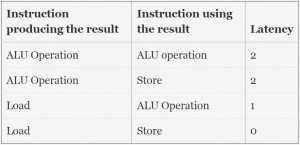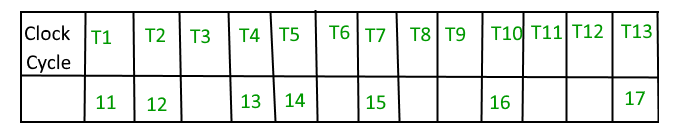# GATE | Gate IT 2007 | Question 41

Following table indicates the latencies of operations between the instruction producing the result and instruction using the result.`Load R1, Loc 1;     Load R1 from memory location Loc1 ` `Load R2, Loc 2;     Load R2 from memory location Loc 2 ` `Add R1, R2, R1;     Add R1 and R2 and save result in R1 ` `Dec R2;     Decrement R2 ` `Dec R1;     Decrement R1 ` `Mpy R1, R2, R3;     Multiply R1 and R2 and save result in R3 ` `Store R3, Loc 3;     Store R3 in memory location Loc 3 `

What is the number of cycles needed to execute the above code segment assuming each instruction takes one cycle to execute ?
(A) 7
(B) 10
(C) 13
(D) 14

Explanation:

In the given question there are 7 instructions each of which takes 1 clock cycle to complete. (Pipelining may be used)
If an instruction is in execution phase and any other instructions can’t be in the execution phase. So, atleast 7 clock cycles will be taken.
Now, it is given that between two instructions latency or delay should be there based on their operation. Ex- 1st line of the table says that between two operations in which first is producing the result of an ALU operation and the 2nd is using the result there should be a delay of 2 clock cyles.

clock cycle :3) Add R1, R2, R1; Add R1 and R2 and save result in R1
R1=R1+R2;

Hence, this instruction is using the result of R1 and R2, i.e. result of Instruction 1 and Instruction 2.

As instruction 1 is load operation and instruction 3 is ALU operation. So, there should be a delay of 1 clock cycle between instruction 1 and instruction 3.Which is already there due to I2.
As instruction 2 is load operation and instruction 3 is ALU operation. So, there should be a delay of 1 clock cycle between instruction 2 and instruction 3.

4) Dec R2; Decrement R2
This instruction is dependent on instruction 2 and there should be a delay of one clock cycle between Instruction 2 and Instruction 4. As instruction 2 is load and 4 is ALU . Which is already there due to Instruction 3.

5) Dec R1 Decrement R1
This instruction is dependent on Instruction 3
As Instruction I3 is ALU and I5 is also ALU so a delay of 2 clock cycles should be there between them of which 1 clock cycle delay is already there due to I4 so one clock cycle delay between I4 and I5.

6) MPY R1, R2, R3; Multiply R1 and R2 and save result in R3
R3=R1*R2;
This instruction uses the result of Instruction 5, as both instruction 5 and 6 are ALU so there should be a delay of 2 clock cycles.

7) Store R3, Loc 3 Store R3 in memory location Loc3
This instruction is dependent on instruction 6 which is ALU and instruction 7 is store so there should be a delay of 2 clock cycles between them.

Hence, a total of 13 clock cycles will be there.

This solution is contributed by Shashank Shanker khare.

Quiz of this Question

My Personal Notes arrow_drop_up
Article Tags :

Be the First to upvote.

Please write to us at contribute@geeksforgeeks.org to report any issue with the above content.